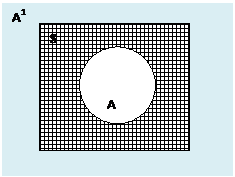# www.campusplace.co.in

One stop blog for all competitive examinations

Comparability of Sets:   Two sets A and B are said to be comparable if either of these happens: (i)           A Ì B (ii)        ...

# Aptitude for Placement Papers - Set Theory -2

Comparability of Sets:
Two sets A and B are said to be comparable if either of these happens:
(i)          A Ì B
(ii)         Ì A
(iii)        A = B
Similiarly if neither of these above three exisits i.e., A Ë B, B Ë A and A≠B, then let A and B are said to be incomparable.
Ex:  A={1,2,3} and B={1,2}
Hence set A and B are comparable.
But A ={1, 2, 3} and B={2, 3, 6, 7} are incomparable.

Univerasal Set:
Any set which is super set of all the sets under consideration is known as the universal set and is either denoted by Ω or S or U.
Ex: Let A ={ 1, 2, 3} and B={3, 4,6, 9} and C={0, 1}
We can take, S={0, 1, 2, 3, 4, 5, 6, 7, 8, 9} and Universal Set.

Power Set:
The set or family of all the subsets of a given set A is said to be the power of A and is expressed by P(A).
Note:
(i)          ØP(A) and AP(A) for all sets A.
(ii)         The elements of P(A) are the subsets of A.
Ex: If, A = {1}, then P(A)+{Ø,{1}}
If A {1, 2}, then P(A)= {Ø, {1}, {2}, {1, 2}}.
Similarly if A={1, 2, 3}, then P(A)={Ø, {1}, {2}, {3}, {1, 2}, {1, 3}, {2, 3}, {1, 2, 3}}
So trends, show that if A has n elements then P(A) has 2n elements. Further to note that it is not only true for n=1, 2, 3, but it is true of all n.

Some Operations on Sets:
Union of Sets:
The union of two sets A and B is set of all those elements which are either in A or in B or in both. This set is denoted by A U B and read as ‘A union B’.
Symbolically, A È B ={x : xA or xB}
Or                a È b ={x: xA U xB}
Example : A= {1,3,5,7,9}  B= { 2,4,5,6,7} then A È B = { 1,2,3,4,5,6,7,9 }

Intersection of Sets:
The intersection of two sets A and B is the set of all the elements, which are common in A and B. This set is denoted by A (intersection) B and read as ‘A intersection of B’. i.e.,
A Ç B={x: xA and xB}
Or A Ç B ={x: xA Ç xB}
Example : A= {1,3,5,7,9}  B= { 2,4,5,6,7} then A Ç B = { 5,7}

Difference of Sets:
The difference of two sets A and B, is the set of all those elements of A which are not elemtns of B. Sometimes, we call differeence of sets as the relative components of B in A. It is denoted by A (Intersection)B.
i.e.,    A-B ={x: xA and xB}
Similary B-A={x: xB and xA}
Example : A={ 1,3,5,6,8,10}  B={ 2,3,4,5,6,7}, the A-B = {1,8,10}

Symmetric Difference of Sets:
If A and B are two sets then A ∆ B =(A-B) È (B-A) or (AÈB) – (A Ç B) is called Symmetric Difference of Sets.

Complements of Set:
The complement of a set A, also known as absolute complement of A is the sets of all those element of the universal sets which are not element of A. It is denoted by Ao or A-1.
Infact           A1 or Ac = U – A.
Or                A1= {x: xU and xA}.

Properties:
If A, B, C are three sets, then
(i)          Idempotent laws: AÈ A=A, AÇ A=A.
(ii)         Commutative laws: A È B=B È A, A Ç B = B Ç A.
(iii)        Associative laws: (A È B)È C = AÈ(BÈC); (AÇ B)Ç C = A Ç (B Ç C).
(iv)        Identity Laws: AUØ=A; AÇØ=Ø; AUµ=µ; AÇ µ=A.
(v)         Complement laws: AUA1=µ; AÇA1=Ø; Ø1=µ,µ1=Ø.
(vi)        Involution law: (A1)1=A.
(vii)      Demorgan laws: A-(BUC) = (A-B) inttersection (A-C);
A-(BÇC)=(A-B) U(A-C);
(AUB)1=A1Ç B1; (AÇ B)1=A1UB1
(viii)     Distributive laws: AU(BÇ C) =(AUB)Ç (AUC);
A Ç (BUC) = (A Ç B) U (A  Ç C).

Some more important results:
i)             AÈB=Ø ó A=Ø and B=Ø.
ii)           A-B=Ø ó A Í B
iii)          A Ì A È B; B Ì A È B; A Ç B Ì A; A Ç B Ì B.
iv)          A-B≠B-A; A-BÌA; A-B= AÇ B1; A-B = A ∆ (AÈB).
v)           A Ì B ó B1Ì  A1
vi)          n(AÈB)= n(A)+n(B)-n(A intersection B).
vii)         n(AÈBÈC)= n(A)+n(B)+n(C) – n(A Ç B) – n(B Ç C) – n(C Ç A) + n(A Ç B Ç C).

VENN DIAGRAM AND SOME OF THE APPLICATIONS OF SET THEORY.
Venn diagram is a pictorial representation of sets.
A set is represented by circle or a closed geometrial figure inside the universal set.
The Universal Set S, is represented by a rectangular region. First of all we will represent the set or a statmenet regarding sets with the help of the diagram or Venn Diagram. The shaded area represents the set written.

(a) Subset:       A Ì B

(b) Union of Sets:  Let A U B = B. Here, whole area represented by B represents A U B.

A U B when A Ì B

U B when neither   A ÌB nor BÌA

AÈB when A and B are disjoint events

(c) Intersection of Sets:  A (intersection) B represents the common area of A and B.

AÇB when A Ì B , (AÇB=A)

AÇB when neither A Ì B nor B Ì A

(AÇB=Ø) when A and B are disjoint sets.

(a) Difference of Sets: (A-B).
A-B represents the area of A that is not in B.

A-B when AÌB (A-B=Ø)

A-B when BÌA

A-B neither A Ì B nor B Ì A

e) Complement of Sets:
A1 or Ac is the set of those points of Universal set S which are not in A.